## 13.2 Bar plots

A bar chart or bar plot displays rectangular bars with lengths proportional to the values that they represent.

• A simple bar plot :
``````# Create a vector
mycenter <- rep(x=c("PhDstudent", "Postdoc", "Technician", "PI"),
times=c(8,10,5,2))

# Count number of occurrences of each character string
mytable <- table(mycenter)

# Bar plot using that table
barplot(height=mytable)``````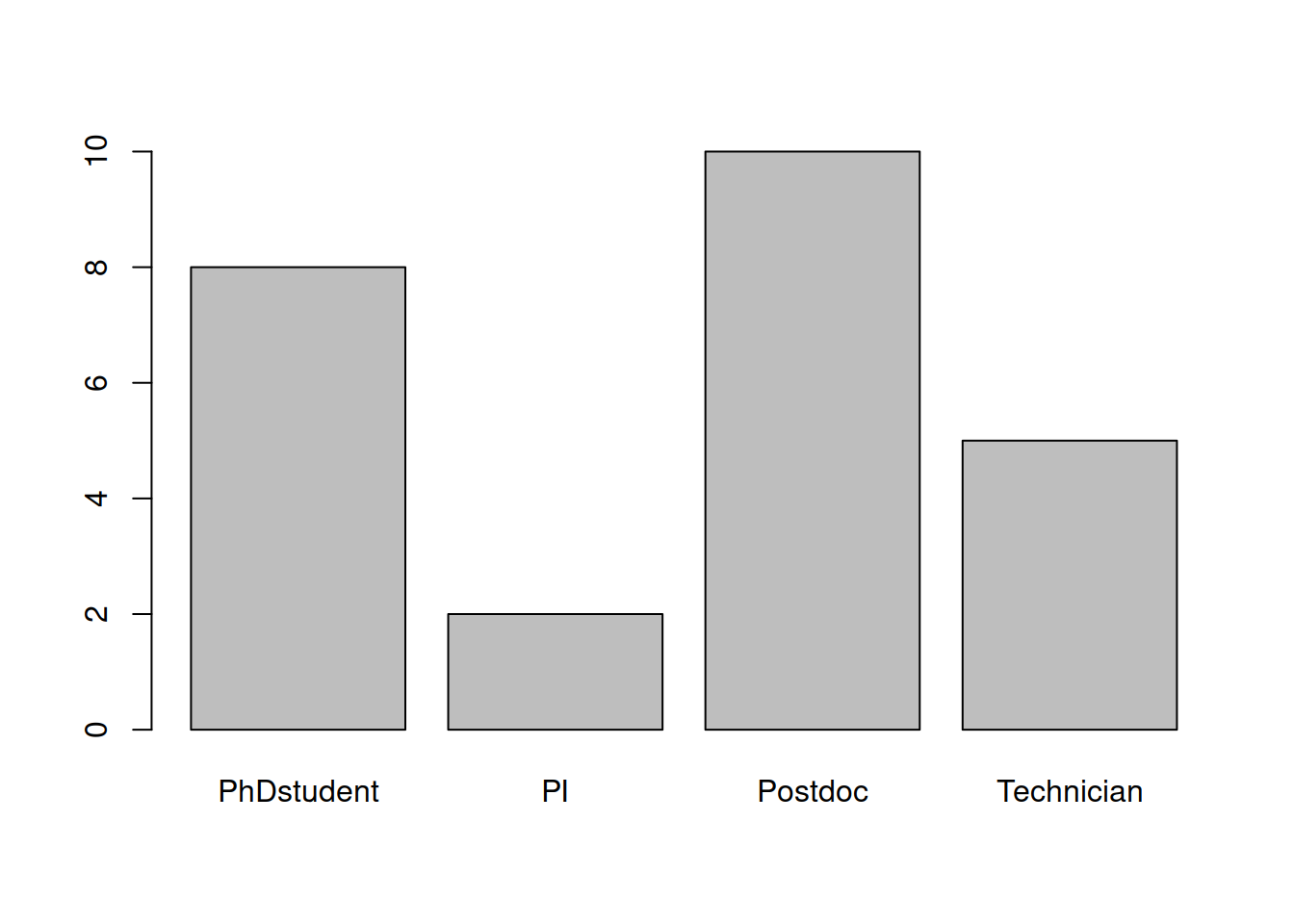• Customize a bit :
• col : color
• main : title of the plot
• las : orientation of axis labels:
• 0: all labels parallel to axis
• 1: x-axis labels parallel / y-axis labels perpendicular
• 2: both labels perpendicular
• 3: x-axis labels perpendicular / y-axis labels parallel
``````barplot(height=mytable,
col=1:4,
main="bar plot",
las=2)``````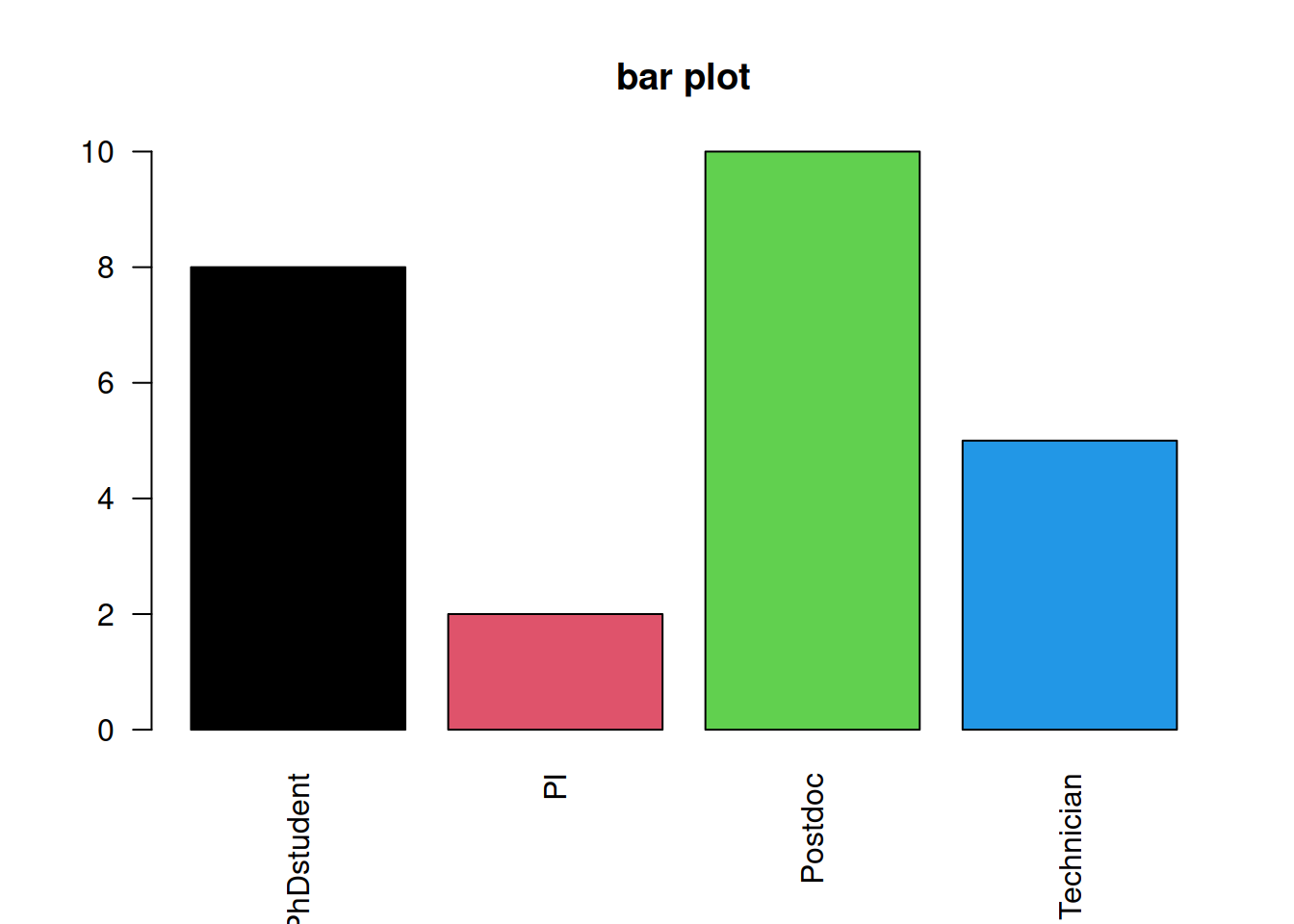• Customize the ordering of the bars :

By default, the bars are organized in alphabetical order. You can change it using an ordered factor.

``````# Create an ordered factor out of mycenter: the order in which you write the "levels" is the sort in which the bars will next be plotted
xfact <- factor(x=mycenter,
levels=c("PhDstudent", "Postdoc", "Technician", "PI"),
ordered=TRUE)

# Produce the table
xfacttable <- table(xfact)

# Plot the same way
barplot(height=xfacttable,
col=1:4,
main="reorganized bar plot",
las=2)``````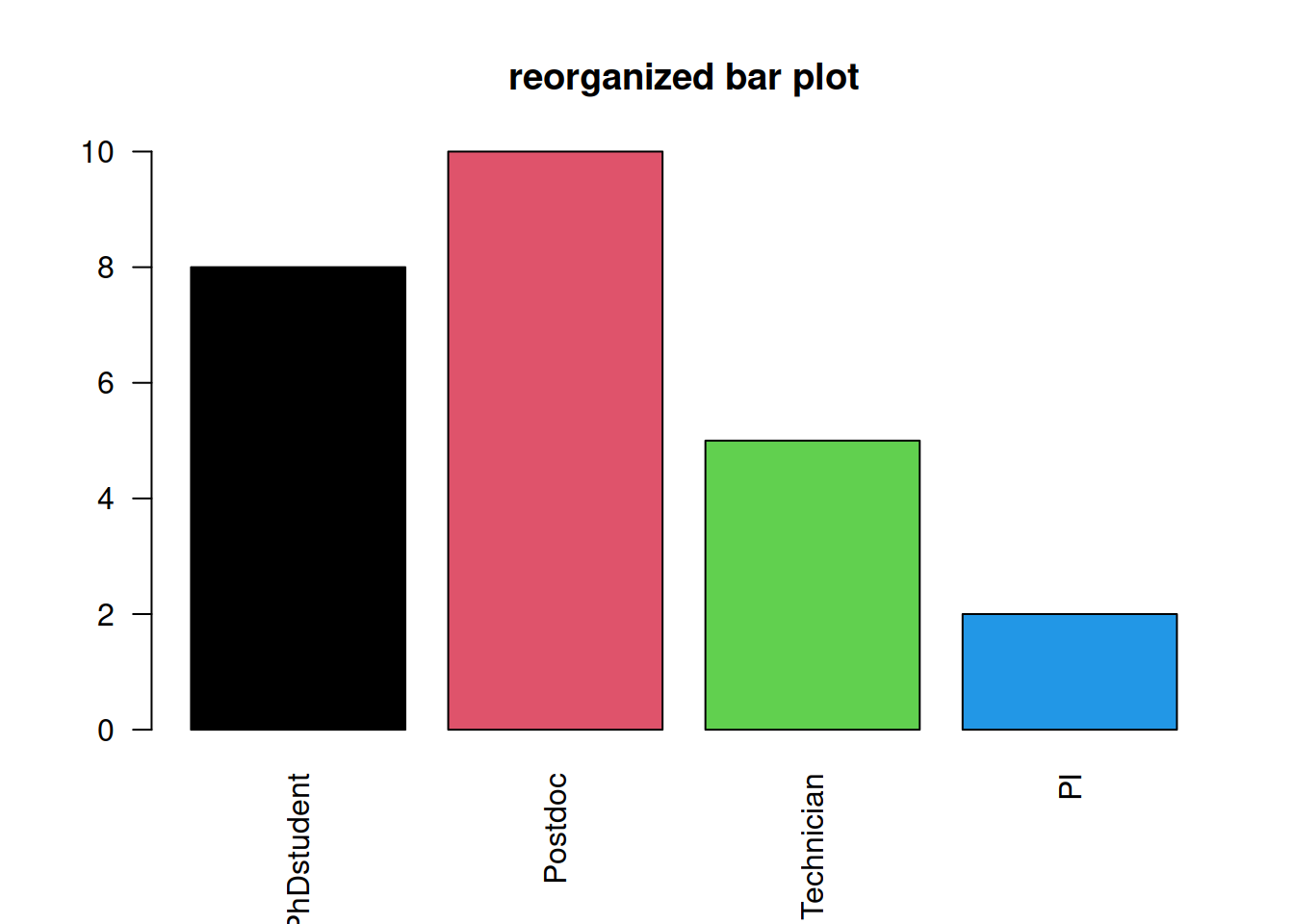• We can also produce stacked barplot :
``````# Create a matrix containing the number and type of employees per research program :
barmat <- matrix(c(8, 10, 9, 2, 6, 4, 5, 3, 14, 13, 16, 4, 11, 10, 8, 5),
nrow=4,
dimnames=list(c("Technician", "PhDstudent", "PostDoc", "PI"), c("BG", "CDB", "GRSCC", "SB")))

# Plot barplot
barplot(height=barmat,
col=sample(colors(), 4))``````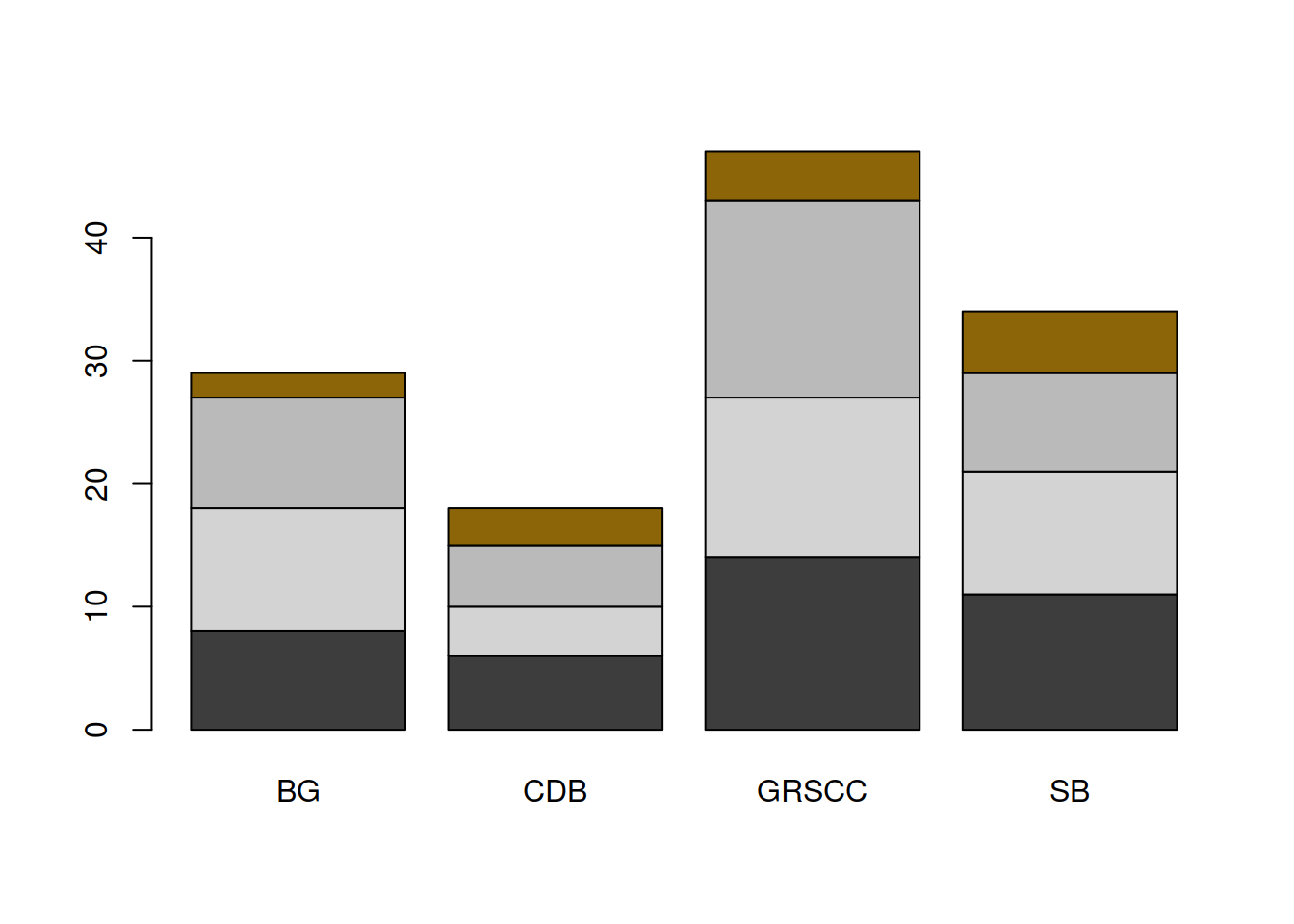``````# set a random color vector
# add set.seed(38) (or any other number) to reproduce the randomization.
mycolors <- sample(x=colors(),
size=4)

# plot barplot
# ylim sets the lower and upper limit of the y-axis: here it allows us to fit the legend !
barplot(height=barmat,
col=mycolors,
ylim=c(0,50),
main="stacked barplot")``````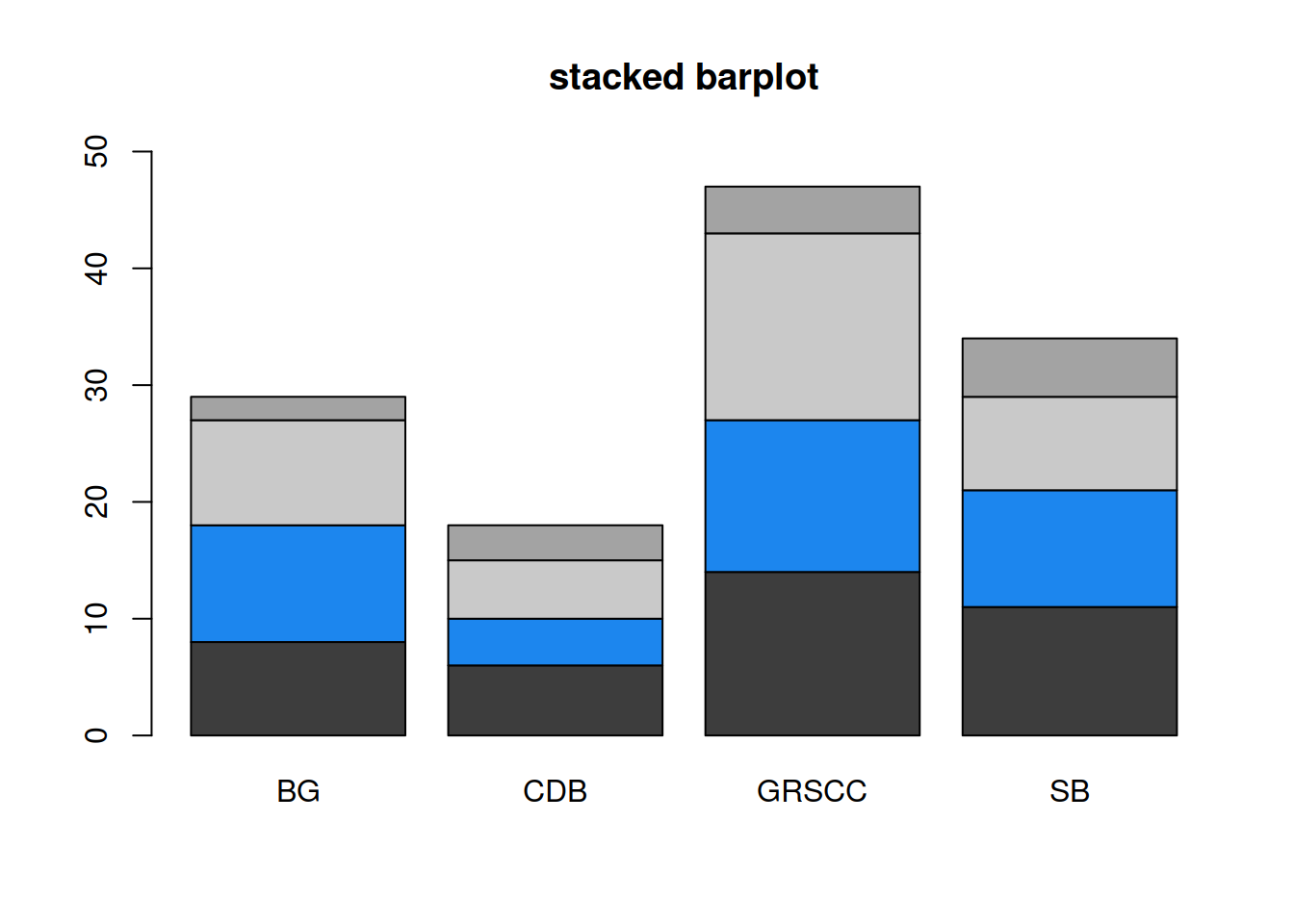• Add a legend to the plot:
• “x” and “y” set the legend’s position in the plotting area: you can specify the position as coordinates using “x” and “y.”
• if “x” only is used, you can set the legend position as “topleft,” “bottomleft,” “topright,” “bottomright”
• Note: `barplot()` (or any other plot function) has to be called first
``````barplot(height=barmat,
col=mycolors,
ylim=c(0,50),
main="stacked barplot")

legend(x="topleft",
legend=c("Technician", "PhDstudent", "PostDoc", "PI"),
fill=mycolors)``````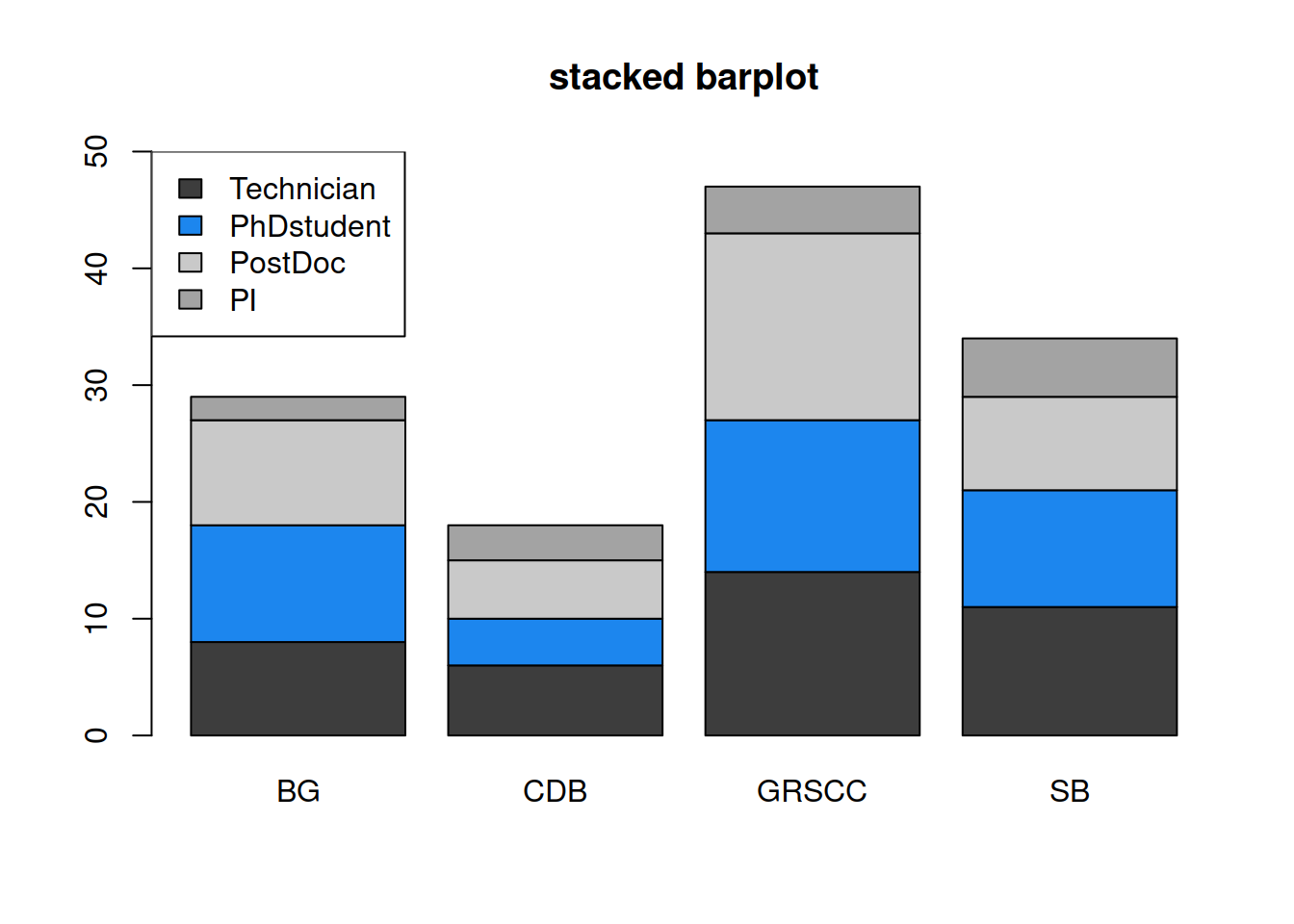A more automated way to do this:

``````legend(x="topleft",
legend=rownames(barmat),
fill=mycolors)``````

HANDS-ON

The dataset chickwts is also a built-in dataset from the `datasets` package: the table measures and compares the effectiveness of various feed supplements on the growth rate of chickens.

• Create a barplot of the different feed supplements.
• Change the orientation of the x-axis labels.
• Try to re-organize the bars by the increasing number of feed supplements.
``````# Create a barplot of the different **feed supplements**.
tablefeed <- table(chickwts\$feed)
barplot(tablefeed)

# Change the orientation of the x-axis labels.
barplot(tablefeed, las=2)

# Try to re-organize the bars by the increasing number of feed supplements.
# check tablefeed and write the feed categories in increasing order:
feedfactor <- factor(x=chickwts\$feed, levels=c("horsebean", "meatmeal", "casein", "linseed", "sunflower", "soybean"),
ordered=TRUE))

# a less "manual" way to proceed: tablefeed (the output of table() ) is a NAMED vector: sort it and retrieve its names in sorted order
sort(tablefeed)
names(sort(tablefeed))
feedfactor <- factor(x=chickwts\$feed,
levels=names(sort(tablefeed)),
ordered=TRUE)

# plot sorted barplot
barplot(table(feedfactor), las=2)``````